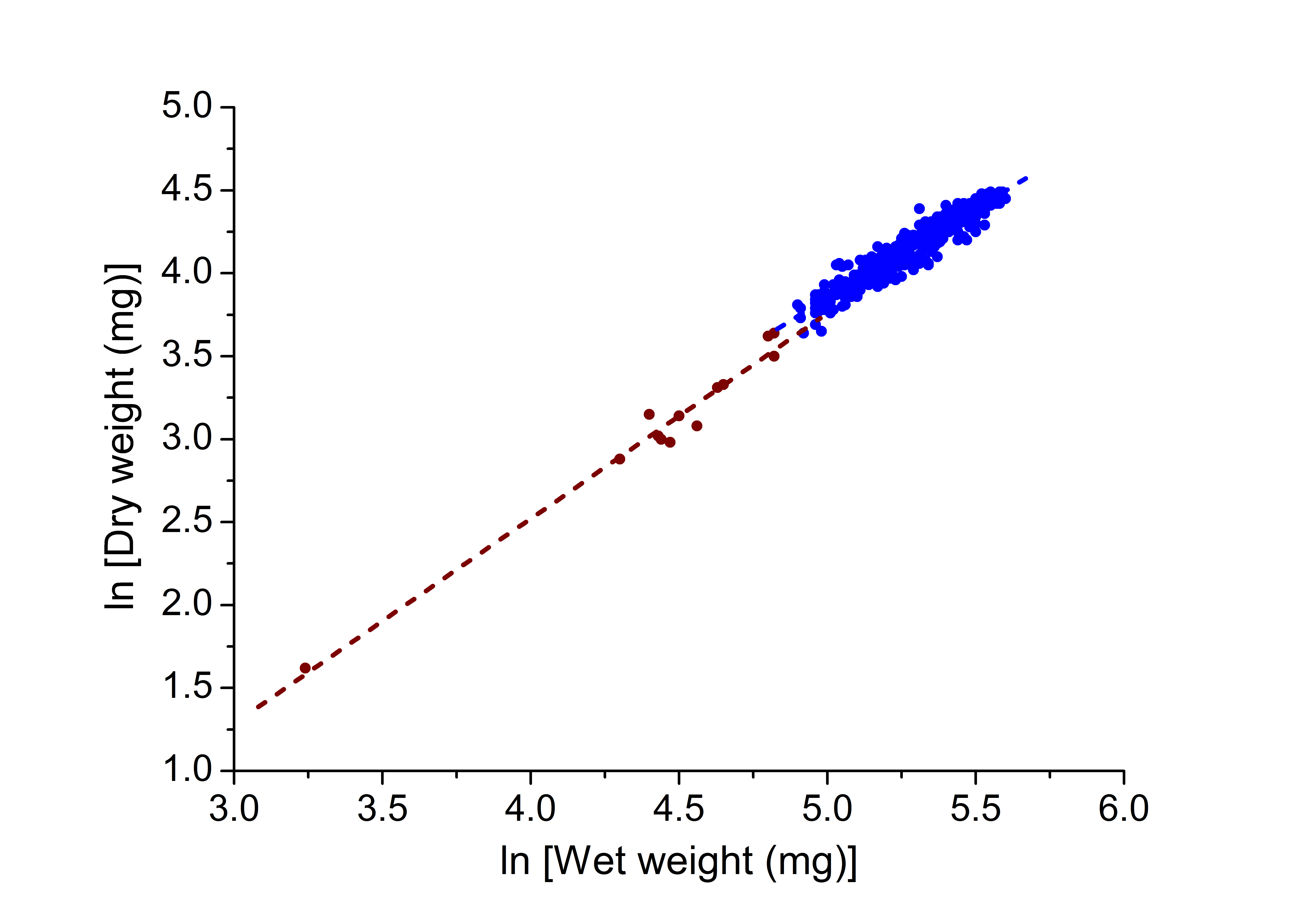Figure 3 of Mohamed, Mol Vis 2018; 24:867-874.

Figure 3. Allometric analysis of the relationship between wet and dry weights. Data from all lenses less than 2 years old can be described with the equation ln [dry weight] =2 .42 (± 0.28) + 1.24 (± 0.06) × ln [wet weight] (R2=0.97; p<0.0001; n=13); for all ages 3–93, the equation is ln [dry weight] = 1.56 (± 0.08) + 1.08 (± 0.02) × ln [wet weight] (R2=0.91; p<0.0001; n=480).